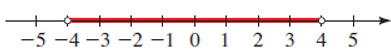# an inequality involving an absolute value that describes the sets.### Precalculus: Mathematics for Calcu...

6th Edition
Stewart + 5 others
Publisher: Cengage Learning
ISBN: 9780840068071### Precalculus: Mathematics for Calcu...

6th Edition
Stewart + 5 others
Publisher: Cengage Learning
ISBN: 9780840068071

#### Solutions

Chapter 1.7, Problem 96E
To determine

## To find:an inequality involving an absolute value that describes the sets.

Expert Solution

|x|<4 .

### Explanation of Solution

Given:

A set of real number is graphed on the number line:Concept used:

Properties of absolute value inequalities:

 Inequality Equivalent form Graph |x|c x<−c or c

Calculation:

According to the properties of absolute value inequalities, theequivalent form of the given set of real number is 4<x<4

Therefore, the inequality is |x|<4 .

### Have a homework question?

Subscribe to bartleby learn! Ask subject matter experts 30 homework questions each month. Plus, you’ll have access to millions of step-by-step textbook answers!